# MP Board Class 9th Science Solutions Chapter 4 Structure of the Atom

## MP Board Class 9th Science Solutions Chapter 4 Structure of the Atom

### Structure of the Atom Intext Questions

Structure of the Atom Intext Questions Page No. 47

Question 1.
What are canal rays?
Canal rays are positively charged radiations which led to the discovery of positively charged sub-atomic particle called proton. These rays were discovered by E. Goldstein.Question 2.
If an atom contains one electron and one proton, will it carry any charge or not?
The atom will not contain any charge and will be electrically neutral because both electron and proton will balance each other.

Structure of the Atom Intext Questions Page No. 49

Question 1.
On the basis of Thomson’s model of an atom, explain how the atom is neutral as a whole.
According to Thomson’s model, an atom consist of a positively charged sphere and electrons are embedded in it. So, both charges are equal which makes the atom electrically neutral.

Question 2.
On the basis of Rutherford’s model of an atom, which sub – atomic particle is present in the nucleus of an atom?
Proton is the sub – atomic particle which is present in the nucleus of an atom.Question 3.
Draw a sketch of Bohr’s model of an atom with three shells.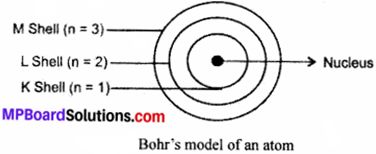Question 4.
What do you think would be the observation if the a-particle scattering experiment is carried out using a foil of a metal other than gold?
The observations would be same as that of gold foil.

Structure of the Atom Intext Questions Page No. 49

Question 1.
Name the three sub – atomic particles of an atom.

1. Positively charged – Protons
2. Negatively charged – Electrons
3. No charged – Neutrons.

Question 2.
Helium atom has an atomic mass of 4u and two protons in its nucleus. How many neutrons does it have?
Atomic mass = Number of protons + Number of neutrons
∴ 4 = 2 + Number of neutrons
∴ Number of neutrons = 4 – 2 = 2.

Structure of the Atom Intext Questions Page No. 50

Question 1.
Write the distribution of electrons in carbon and sodium atoms.
Atomic number of Carbon = 6Question 2.
If K and L shells of an atom are full, then what would be the total number of electrons in the atom?
K shell is the 1 shell
So, n = 1
Then maximum electron’s = 2n2 = 2 × (1)2
= 2 × 1 = 2
and L shell is the second shell.
So, n = 2
Then maximum electrons = 2(n)2
= 2 × (2)2 = 8
∴ Total number of electrons = 2 + 8 = 10.

Structure of the Atom Intext Questions Page No. 52

Question 1.
How will you find the valency of chlorine, sulphur and magnesium?
We know that valency is the number of electrons lost, gained or shared by atom to become stable or to complete 8 electrons in the shell.
Now, Chlorine,
Atomic number = 17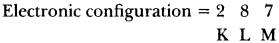Then, it will take 8 – 7 = 1 electron to complete its shell.
∴ Its valency is ‘I’
Sulphur, Atomic number = 16It will take 8-6 = 2 electrons to complete its shell.
∴ Its valency is ‘2’.
Magnesium, Atomic number = 12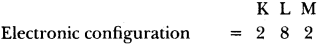It will lose 2 electrons from its outermost shell to become stable.
∴ Its valency will be ‘2’.

Structure of the Atom Intext Questions Page No. 52

Question 1.
If number of electrons in an atom is 8 and number of protons is also 8 then,
(i) What is the atomic number of the atom? and
(ii) What is the charge on the atom?
(i) Number of electrons = 8 and,
Number of protons =8
Then, Atomic number = Number of protons = 8

(ii) Now, total electrons (-) = Total protons (+)
So, atom will be electrically neutral.Question 2.
With the help of table 4.1 of Textbook, find out the mass number of oxygen and sulphur atom.
From the table, we have,
Oxygen,
Mass Number = Number of protons + Number of neutrons
= 8 + 8 = 16
Sulphur,
Mass number = Number of protons + Number of neutrons
= 16 + 16 = 32.

Structure of the Atom Intext Questions Page No. 53

Question 1.
For the symbol H, D, and T tabulate three sub – atomic particles found in each of them.
H, D, and T stand for protium, deuterium and tritium as isotopes of hydrogen atom.
Table: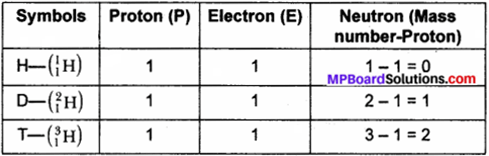Question 2.
Write the electronic configuration of any one pair of isotopes and isobars.
Pair of isotopes: $$_{ 6 }^{ 12 }{ C }$$, $$_{ 6 }^{ 14 }{ C }$$
Electronic configuration:### Structure of the Atom NCERT Textbook Exercises

Question 1.
Compare the properties of electrons, protons and neutrons.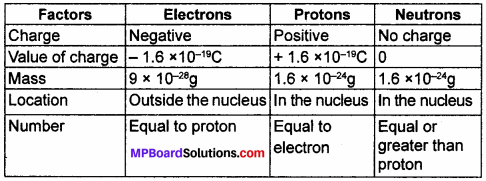Question 2.
What are the limitations of J.J. Thomson’s model of the atom?
J.J. Thomson’s model explained the existence of positive charge in the form of sphere and electrons embedded in it. But, he was unable to explain the Rutherford’s gold foil experiment in which most of positive α – particles passed straight, existence of electrons in the circular path and protons at the centre of the atom.Question 3.
What are the limitations of Rutherford’s model of the atom?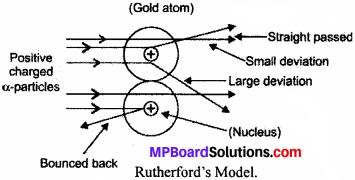Rutherford explained that electrons revolve in a circular path which is found contradictory in terms of stability of atom. Because electrons are negatively charged and when they move continuously in circular paths then they should lose their energies and finally, fall into the positively charged nucleus making atoms unstable and collapse.

Question 4.
Describe Bohr’s Model of the atom.
Neils Bohr proposed the theory for model of the atom. It is explained as:

1. Atom is made up of three sub – atomic particles as electrons, protons and neutrons.2. The electrons move round the nucleus in fixed circular paths called orbits or shells.
3. The orbits are represented by the letters K, L, M, N, or the number n = 1, 2, 3, 4.
4. Centre of the atom is called the nucleus.
5. Electrons do not radiate energies while revolving in the orbits.
6. Electrons gain energy when they jump from lower shell to higher shell and lose energy when they return down from higher energy level to lower energy level.

Question 5.
Compare all the proposed models of an atom given in this chapter.Question 6.
Summarise the rules for writing of distribution of electrons in various shells for the first eighteen elements.
Rules:

(a) Maximum electrons present in a shell is given by 2n2 whereas n is the number of that shell.
Like,

• K Shell, n = 1 → 2n2 = 2 × (1)2 = 2
• L Shell, n = 2 → 2n2 = 2 × (2)2 = 8
• M Shell, n = 3 → 2n2 = 2 × (3)2 = 18
• N Shell, n = 4 → 2n2 = 2 × (4)2 = 32.

(b) The outermost shell can have maximum of 8 electrons.
(c) Electrons cannot be occupied in a shell till its inner shells or orbits are completely filled.

Question 7.
Define valency by taking examples of silicon and oxygen.
Valency is the combining capacity of an atom to become electrically stable. Or It means how many electrons are lost or gained by an atom to become stable.
In Silicon,It has 4 valence electrons.
So, it will lose 4 electrons to become stable.
∴ Its valency is 4.
In Oxygen,It has 6 valence electrons.
So, it will gain 8 – 6 = 2 electrons to become stable.
∴ Its valency is 2.

Question 8.
Explain with examples:
(i) Atomic number
(ii) Mass number
(iii) Isotopes
(iv) Isobars
Give any two uses of isotopes.
(i) Atomic number: It is equal to the total number of
protons in the nucleus of its atom.
E.g.,

• Carbon has 6 protons. So, its atomic number is 6.

(ii) Mass number: It is equal to the sum of total number of protons and neutrons in the nucleus.
E.g.,

• Sodium has 11 protons and 12 neutrons. So, its mass number is 11 + 12 = 23

(iii) Isotopes: These are atoms of the same element having same atomic number, but different mass number.
E.g.

• $$_{ 35 }^{ 79 }{ Br }$$, $$_{ 35 }^{ 81 }{ Br }$$, $$_{ 6 }^{ 12 }{ C }$$, $$_{ 6 }^{ 14 }{ C }$$

(iv) Isobars: These are the atoms of different elements having different atomic number but same mass number.
E.g.

• $$_{ 18 }^{ 40 }{ Ar }$$, $$_{ 20 }^{ 40 }{ Ca }$$, $$_{ 11 }^{ 24 }{ Na }$$, $$_{ 12 }^{ 24 }{ Mg }$$

Use of Isotopes:

• Uranium isotope is used as a fuel in nuclear reactor for generating electricity.
• Sodium isotope is used to detect the blood clots.Question 9.
Na+ has completely filled K and L shells. Explain.
Atomic number of sodium (Na) is 11.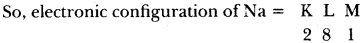Now, if Na loses 1 electron then it will become Na+.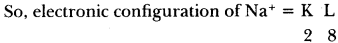Now K shell can have maximum of 2 electrons and L shell can have maximum of 8 electrons.
Then, Na+ has completely filled K and L shell.

Question 10.
If bromine atom is available in the form of, say, two isotopes $$_{ 35 }^{ 79 }{ Br }$$ (49.7%) and $$_{ 35 }^{ 81 }{ Br }$$Br (50.3%), calculate the average atomic mass of bromine atom.
Average atomic mass of bromine atom
= 49.7% of atomic mass of $$_{ 35 }^{ 79 }{ Br }$$ + 50.3% of atomic mass of $$_{ 35 }^{ 81 }{ Br }$$
= 49.7% of 79 + 50.3% of 81
= $$\frac { 49.7 }{ 100 }$$ × 49 + $$\frac { 450.3 }{ 100 }$$ × 81
= (39.263 + 40.743)u = 80.006u

Question 11.
The average atomic mass of a sample of an element X is 16.2u. What are the percentages of isotopes If $$_{ 8 }^{ 16 }{ X }$$ and $$_{ 8 }^{ 18 }{ X }$$ in the sample?
Let the percentage of $$_{ 8 }^{ 16 }{ X }$$ in sample be x% and percentage of $$_{ 8 }^{ 18 }{ X }$$ in sample be (100 – x)%.
Now,
x% of 16 + (100 – x)% of 18 = 16.2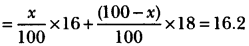-2x + 1800 = 16.2 × 100 – 2x + 1800 = 1620
∴ -2x = 1620- 1800 = -180
x = $$\frac {180}{2}$$ = 90.
∴ Percentage of  $$_{ 8 }^{ 16 }{ X }$$ is 90% and percentage of $$_{ 8 }^{ 18 }{ X }$$ is (100 – 90)% = 10%.

Question 12.
If Z = 3, what would be the valency of the element? Also, name the element.
Z = 3
So, atomic number = 3 (∵ Z = atomic number)
∴ Electronic configuration = 2, 1
Valency = 1
The name of the element is lithium (Li).

Question 13.
Composition of the nuclei of two atomic species X and Y are given as underGive the mass number of X and Y. What is the relation between the two species?
Mass number of X = Protons + Neutrons = 6 + 6 = 12
And,
Mass number of Y = Protons + Neutrons = 6 + 8 = 14
Both species have same atomic number.
So, they are isotopes of the same element.

Question 14.
For the following statements, write T for True and F for False.

1. J.J. Thomson proposed that the nucleus of an atom contains only nucleons.
2. A neutron is formed by an electron and a proton combining together. Therefore, it is neutral.
3. The mass of an electron is $$\frac {1}{2000}$$ times that of proton.
4. An isotope of iodine is used for making tincture iodine which is used as a medicine.

1. False
2. False
3. True
4. False.

Put tick (✓) against correct choice and cross (✗) against wrong choice in questions 15, 16 and 17.

Question 15.
Rutherford’s alpha – particle scattering experiment was responsible for the discovery of.
(a) Atomic nucleus
(b) Electron
(c) Proton
(d) Neutron.
(a) Atomic nucleus

Question 16.
Isotopes of an element have.
(a) the same physical properties
(b) different chemical properties
(c) different number of neutrons
(d) different atomic numbers.
(c) different number of neutronsQuestion 17.
Number of valence electrons in Cl ion are:
(a) 16
(b) 8
(c) 17
(d) 18
(b) 8

Question 18.
Which one of the following is a correct electronic configuration of sodium?
(a) 2, 8
(b) 8, 2, 1
(c) 2, 1, 8
(d) 2, 8, 1.
(d) 2, 8, 1.

Question 19.
Complete the following table:

 Atomic Number Mass Number Number of Neutrons Number of Protons Number of Electrons Name of the Atomic Species 9 – 10 – – – 16 32 – – – Sulphur – 24 – 12 – – – 2 – 1 – – – 1 0 1 0 –

 Atomic Number Mass Number Number of Neutrons Number of Pro-­tons Number of Elec­trons Name of the Atomic Species 9 19 10 9 9 Fluorine 16 32 16 6 6 Sulphur 12 24 12 12 12 Magnesium 1 2 1 1 1 Hydrogen 1 1 0 1 0 Deuterium

### Structure of the Atom Additional Questions

Structure of the Atom Multiple Choice Questions

Question 1.
Which is a positive sub – atomic particle?
(a) Proton
(b) Neutron
(c) Electron
(d) None of these.
(a) Proton

Question 2.
Electron is discovered by _____ .
(b) Neils Bohr
(c) J.J Thomson
(d) Rutherford.
(c) J.J Thomson

Question 3.
Proton is discovered by _____ .
(a) Rutherford
(c) J J. Thomson
(d) E. Goldstein.
(d) E. Goldstein.

Question 4.
Neutron is discovered by _____ .
(a) J.J. Thomson
(c) Neils Bohr
(d) Rutherford.

Question 5.
Nucleus is discovered by _____ .
(a) Rutherford
(c) J.J. Thomson
(d) Neils Bohr.
(a) RutherfordQuestion 6.
Mass of electron is _____ .
(a) 9 × 10-25g
(b) 6 × 10-28g
(c) 8 × 10-24g
(d) 9 × 10-28g.
(d) 9 × 10-28g.

Question 7.
Mass of Neutron is _____ .
(a) 1.6 × 10-22g
(b) 1.6 × 10-23g
(c) 1.6 × 10-25g
(d) 1.6 × 10-24g.
(d) 1.6 × 10-24g.

Question 8.
Charge on an electron is _____ .
(a) -1.8 × 10-18C
(b) -1.7 × 10-20C
(c) -1.6 × 10-19C
(d) -1.5 × 10-21C.
(c) -1.6 × 10-19C

Question 9.
The energy paths in an atom in which electrons revolve are called _____ .
(a) Rings
(b) Cycles
(c) Orbits
(d) Circles.
(c) Orbits

Question 10.
ass number is the sum of _____ .
(a) Protons and Electrons
(b) Protons and Neutrons
(c) Electrons, Protons and Neutrons
(d) None of these.
(c) Electrons, Protons and Neutrons

Question 11.
Atomic number is equal to _____ .
(a) Number of protons
(b) Number of neutrons
(c) Number of electrons
(d) Both (a) and (c).
(d) Both (a) and (c)

Question 12.
Maximum number of electrons that can be filled in ‘M’ shell are _____ .
(a) 17
(b) 19
(c) 18
(d) 20.
(c) 18

Question 13.
An atom has atomic number ‘17’, then its valency will be _____ .
(a) 7
(b) 2
(c) 1
(d) 8.
(c) 1

Question 14.
Isotopes of an element have same number of _____ .
(a) Neutrons
(b) Protons
(c) Electrons
(d) Both (b) and (c).
(c) Electrons

Question 15.
Isobars of different elements have same _____ .
(a) Atomic number
(b) Electrons
(c) Mass number
(d) Neutrons.
(d) Neutrons

Structure of the Atom Very Short Answer Type Questions

Question 1.
Who discovered canal rays?
E. Goldstein.

Question 2.
Name the fruit which resembles J.J. Thomson model of atom.
Watermelon.

Question 3.
Who discovered nucleus?
Ernest Rutherford.

Question 4.
Who discovered neutrons?

Question 5.
Name the central part of an atom where protons and neutrons are held together.
Nucleus.Question 6.
What is Alpha Particle?
It is a Helium ion (He2+) which has 2 units of positive charge and 4 units of mass.

Question 7.
What are cathode rays?
Cathode rays are a beam of fast moving electrons.

Question 8.
What was the main drawback of Rutherford’s model of the atom?
Inability to explain the stability of atom.

Question 9.
Write the symbolic representation of an element A with atomic number 10 and mass number 20.
$$_{ 10 }^{ 20 }{ A }$$

Question 10.
Name three Isotopes of Hydrogen.

1. Protium ($$_{ 1 }^{ 1 }{ H }$$)
2. Deuterium ($$_{ 1 }^{ 2 }{ H }$$)
3. Tritium ($$_{ 1 }^{ 3 }{ H }$$)

Question 11.
Write the electronic configuration of potassium (K).
Atomic number of potassium (K) =19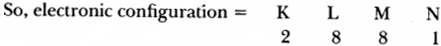Question 12.
Define valency.
It is the combining capacity of an atom to become electrically stable.

Question 13.
What is the charge of a proton?
1.6 × 10-19C.

Question 14.
Write the year of discoveries of these sub – atomic particles – electron, proton, neutron and neucleus.

1. Electron – 1897
2. Proton – 1866
3. Neutron – 1932
4. Nucleus – 1911.

Question 15.
Which radioactive Isotope is used in treatment of goitre?
Iodine – 131.

Structure of the Atom Short Answer Type Questions

Question 1.
Define:
(a) Canal rays
(b) Cathode rays
(c) Atomic number
(d) Mass number
(e) Energy shells
(f) Valency
(g) Octet
(h) Isotopes
(t) Isobars.
(a) Canal rays: These are positively charged radiations which led to the discovery of sub – atomic positively charged particles called protons through an experiment conducted by J.J. Thomson in 1897.

(b) Cathode rays: These are negatively charged radiations which led to the discovery of sub – atomic negatively charged particles called electrons during an experiment conducted by E. Goldstein in 1866.

(c) Atomic number: It is the number of protons present in the nucleus of an atom. It is represented by the letter ‘Z’.

(d) Mass number: It is the total number of protons and neutrons present in an atom of an element.
So, Mass number = Number of protons + Number of neutrons.

(e) Energy Shells: These are fixed circular paths around the nucleus of an atom in which electrons revolve continuously with high speed. These are also called orbits. They are represented by the alphabets K, L, M, N.

(f) Valency: It is the combining capacity of an atom to become electrically stable, or it also means the number of valency electrons lost or gained by an atom to complete the eight electrons in the valence shell.

(g) Octet: The completely filled outermost shell like L, M or N with 8 electrons is called an octet. When an atom completes its octet, then it become stable.

(h) Isotopes: These are atoms of same element having same atomic number, but different mass number.

E.g.

• ($$_{ 1 }^{ 1}{ H }$$) , ($$_{ 1 }^{ 2 }{ H }$$), ($$_{ 1 }^{ 3 }{ H }$$) and $$_{ 6 }^{ 12 }{ C }$$, $$_{ 6 }^{ 14 }{ C }$$are the isotopes of hydrogen and carbon respectively.

(i) Isobars: These are atoms of different elements having different atomic number but same mass number.
E.g.

• $$_{ 18 }^{ 40 }{ Ar }$$, $$_{ 20 }^{ 40 }{ Ca }$$ and $$_{ 11 }^{ 24 }{ Na }$$, $$_{ 12 }^{ 24 }{ Mg }$$.

Question 2.
Differentiate between:
(a) Electrons and protons.
(b) Atomic number and mass number.
(c) Isotopes and isobars.
(d) Valence electrons and valency.
(a)

 Electrons Protons (i) This is negatively charged sub – atomic particle. (i) This is positively charged sub – atomic particle. (ii) Its mass is 9 × 10–28gms. (ii) Its mass is 1.6 × 10-24gms. (iii) Its symbol is “e–”. (iii) Its symbol is “P+”.

(b)

 Atomic number Atomic mass (i) It is the total number of protons present in the atom. (i) It is the sum of protons and neutrons present in the atom. (ii) It is represented by ‘Z’. (ii) It is represented by ‘A’. (iii) It is written on the bottom left as a subscript with the symbol the of element. (iii) It is written on top left as a subscript with the symbol the of element.

(c)

 Isotopes Isobars (i) These are atoms of the same element. (i) These are atoms of the different elements. (ii) They have same atomic number. (ii) They have different atomic number. (iii) They have different mass number. (iii) They have same mass number. (iv) They have same chemical properties. (iv) They have different chemical properties.

(d)

 Valence Electrons Valency (i) These are electrons present in the outermost shell of an atom. (i) These are electrons lost or gained through valence shell of an atom to become stable. (ii) Valence electrons can be 1, 2, 3 …….. 8 or more. (ii) Valency can be 0, 1, 2, 3, 4 only.

Question 3.
Draw the diagrams of:
(a) J.J. Thomson’s model of atom.
(b) Rutherford’s model of atom.
(c) Neils Bohr’s model of atom.
(a) J.J. Thomson’s Model: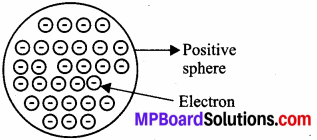(b) Rutherford’s Model:(c) Neils Bohr’s model of atom.Question 4.
Write the postulates of J.J. Thomson’s model of the atom.
J.J. Thomson’s postulates for model of the atom are as follows:

1. An atom is a positively charged sphere or ball and negatively charged electrons are embedded in it.
2. The atom is electrically neutral because negative and positive charges are equal in magnitude.

Question 5.
Write the main points of the theory given by Rutherford for model of atom.
Main points of theory of Rutherford regarding model of atom are:

1. There is an existence of positively charged centre in the atom called as nucleus which contains all the mass of the atom.
2. The electrons revolve round the nucleus in circular paths called orbits at high speeds.
3. The size of nucleus (centre of the atom) is very small as compared to size of the atom.
4. Most of the sphere in an atom is empty.

Question 6.
Write the rules given by Bohr – Bury for arrangement of electrons in different orbits in an atom.
Rules given by Bohr – Bury are as follows:

1. Maximum electrons present in a shell is given by 2n2 where
• n is the number of that shell.
• Like, for first shell K, n = 1
• For second shell L, n = 2
• third shell M, n = 3
• fourth shell N, n = 4 called as nucleus which contains all the mass of the atom.
2. The outermost shell can have maximum of 8 electrons.
3. Electrons cannot occupy a shell till its inner shells or orbits are completely filled.Question 7.
An atom ‘X’ has a mass number ‘23’ and atomic number ‘11’. Find its electrons, protons and neutrons. Also, name the element
We know,
Atomic number = Number of protons.
∴ 11 = Number of protons
And, Number of protons = Number of electrons.
∴ Number of electrons = 11
Now, Mass number = Protons + Neutrons.
23 = 11 + Neutrons
∴ Neutrons = 23 – 11 = 12
∴ Atom ‘X’ has 11 electrons, 11 protons and 12 neutrons.
The element is Sodium (Na).

Question 8.
Write the electronic configuration of neon, aluminium, sulphur, argon. Also, find valencies.Question 9.
What are radioactive isotopes? Write down the type of isotopes used in:
(a) Tracing blood clots and tumours in human body
(b) Treatment of cancer
(c) Treatment of Goitre
(d) Nuclear reactor as a fuel.
Radioactive isotopes: These are unstable isotopes due to extra neutrons in their nucleus and emits different types of radiations.
Examples:

• Uranium – 235
• Cobalt – 60
• Carbon – 14.

Types of Isotopes used in:
(a) Sodium – 24 to detect blood clots and Arsenic – 72 to detect tumours.
(b) Cobalt – 60
(c) Iodine-131
(d) Uranium – 235.

Question 10.
Draw the atomic structure of:
(a) Fluorine atom (F)
(b) Sodium atom (Na)
(c) Potassium atom (K)
(a) Fluorine atom (F):
Atomic number: 9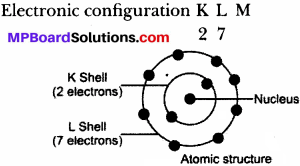(b) Sodium atom (Na)
Atomic number: 11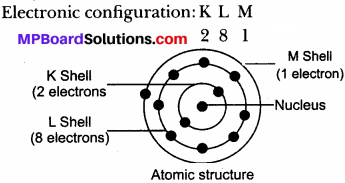(c) Potassium atom (K)
Atomic number: 19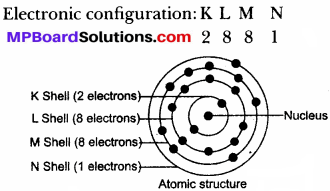Question 11.
Write the atomic number, mass number, electrons, protons and neutrons of following atoms:
(a) $$_{ 14 }^{ 24 }{ X }$$
(b) $$_{ 13 }^{ 27 }{ X }$$
(a) $$_{ 14 }^{ 24 }{ X }$$
Atomic number = 14
Mass number = 24
Electrons = 14
Protons = 14
Neutrons = 24 – 14 = 10

(b) $$_{ 13 }^{ 27 }{ X }$$
Atomic number = 13
Mass number = 27
Electrons = 13
Protons = 13
Neutrons = 27 – 13 = 14

Question 12.
Pick out the Isotopes and Isobars from the following atoms:
$$_{ 17 }^{ 37 }{ A }$$, $$_{ 18 }^{ 40 }{ A }$$, $$_{ 17 }^{ 33 }{ A }$$, $$_{ 20 }^{ 40 }{ A }$$.

1. Isotopes: $$_{ 17 }^{ 37 }{ A }$$, $$_{ 17 }^{ 33 }{ A }$$
2. Isobars: $$_{ 18 }^{ 40 }{ A }$$, $$_{ 20 }^{ 40 }{ A }$$.

Question 13.
What are noble gases? Why they are stable? Give three examples.
Noble gases are the elements which are stable and do not take part in chemical reaction.
They are stable because they have completely filled outer- most shell with 8 electrons
example: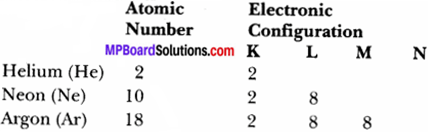Helium is the only noble gas which has 2 electrons in outermost shell.

Question 14.
Write all the Isotopes of:

1. Hydrogen
2. Oxygen
3. Chlorine
4. Bromine
5. Carbon
6. Neon.

1. Hydrogen (H) – $$_{ 1 }^{ 1 }{ H }$$, $$_{ 1 }^{ 2 }{ H }$$
2. Oxygen (O) – $$_{ 8 }^{ 16 }{ O }$$, $$_{ 8 }^{ 17 }{ O }$$, $$_{ 8 }^{ 18 }{ O }$$
3. Chlorine (Cl) – $$_{ 17 }^{ 35 }{ Cl }$$, $$_{ 17 }^{ 37 }{ Cl }$$
4. Bromine (Br) – $$_{ 35 }^{ 79 }{ Br }$$, $$_{ 35 }^{ 81 }{ Br }$$
5. Carbon (C) – $$_{ 6 }^{ 12 }{ C }$$, $$_{ 6 }^{ 14 }{ C }$$
6. Neon (Ne) –  $$_{ 10 }^{ 20 }{ Ne }$$, $$_{ 10 }^{ 21 }{ Ne }$$, $$_{ 10 }^{ 22 }{ Ne }$$

Question 15.
Why is it wrong to say that atomic number of an atom is equal to its number of electrons?
We know that in an atom number of electrons is equal to the number of protons. But, we cannot say that atomic number is equal to number of electrons because number of electrons can be changed after losing or gaining by an atom during chemical reaction. But, number of protons remain constant.

Question 16.
What explanation did Neils Bohr gave on stability of atoms?
Neils Bohr explained the stability of atom through following points:

1. The electrons revolve around the nucleus in fixed orbits or energy levels or shells and each orbit has its fixed radius.
2. While revolving electrons do not radiate their energies, so they do not fall into the nucleus and make the atom stable.

Question 17.
What are nucleons? What is the name given to the atoms having same number of nucleons?
Protons and neutrons together in the nucleus are called nucleons. It means number of nucleons is equal to the sum of protons and neutrons. Atoms having same number of nucleons are called isobars.

Structure of the Atom Long Answer Types Questions

Question 1.
The average atomic mass of a sample of an element X is 13u. What are the percentages of isotopes $$_{ 6 }^{ 12 }{ X }$$ and $$_{ 6 }^{ 14 }{ X }$$ in the sample?
Let, the percentage of isotope $$_{ 6 }^{ 12 }{ X }$$ be x%.
So, percentage of isotope $$_{ 6 }^{ 14 }{ X }$$ is (100- x) %.
Now, Average atomic mass = Mass of $$_{ 6 }^{ 12 }{ X }$$ + Mass of $$_{ 6 }^{ 14 }{ X }$$ According to percentages,
∴ 13 = x% of 12 + (100 – x)% of 14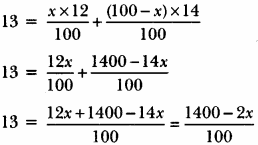∴ 13 × 100 = 1400 – 2x
1300 = 1400 – 2x
1300 = 1400 – 2x
-100 = -2x
x = $$\frac { 100 }{2 }$$ = 50
So, percentage of $$_{ 6 }^{ 12 }{ X }$$ is 50% and percentage of $$_{ 6 }^{ 14 }{ X }$$ is
= (100 – x)%
= (100 – 50)%
= 50%Question 2.
Explain Rutherford’s Gold Foil Experiment. Also, explain its observations conclusion, theory proposed and drawback of his model.
Ernest Rutherford performed an alpha-particles scattering experiment in which he passed a-particles on the gold foil.
Observation:

1. Most of α – particles passed straight without any deflection.
2. Some of the α – particles get deflected from their path.
3. Very few α – particles get completely bounced back.

Conclusions:

1. Maximum space in an atom is vacant as most of α – particles passed straight without any deflection.
2. Some α – particles get deflected from their paths show the existence of positive charge in the atom.
3. Very few α – particles get completely bounced back indicating the concentration of all mass with positive charge in a small volume at the centre.

Theory proposed:

1. There is an existence of positively charged centre in the atom called nucleus which contains all the mass of the atom.
2. The electrons revolve around the nucleus in circular paths called orbits at high speeds.
3. The size of nucleus (centre of the atom) is very small as compared to size of the atom.
4. Most of the space in an atom is empty.

Drawback: Rutherford’s model did not explain the stability of the atom. He proposed that electron revolves around the nucleus in circular paths. So, electrons should radiate their energies as they are continuously in circular motion. Then, they should fall into the positively charged nucleus making the atom unstable and collapse.

Question 3.
Draw the electronic structure of sodium and calcium with atomic number 11 and 20 respectively.
Sodium has electronic distribution as 2, 8, 1
Calcium has electronic distribution as 2, 8, 8, 2
Electronic structures of sodium and calcium are given: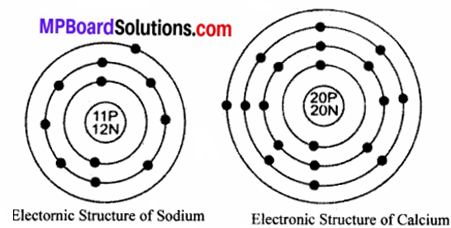Question 4.
Both helium (He) and beryllium (Be) have two valence electrons. Whereas ‘He’ represents a noble gas element, ‘Be’ does not. Assign reason.
The element He (Z = 2) has two electrons present in the only shell
i.e., K – shell. Since, this shell can have a maximum of two electrons only therefore,
‘He’ is a noble gas element.
The element ‘Be’ (Z = 4) has the electronic configuration as: 2,2.
Although, the second shell has also two electrons but it do not represent a noble gas element.

Structure of the Atom Higher Order Thinking Skills (HOTS)

Question 1.
Which isotope of hydrogen contain same number of electrons, protons and neutrons?
Deuterium ($$_{ 1 }^{ 2 }{ D }$$)
Number of electron (1) = Number of proton (1)
= Number of neutron (2 – 1 = 1)

Question 2.
Which element of these two would be chemically more reactive: element A with atomic number 18 or element B with atomic number 16 and why?
Electric configuration of

• A – 2,8,8
• B – 2, 8, 6

Since, the outermost shell of A is complete, it would be inert and will not react. Whereas element B require two atoms to complete its octet. Therefore, B would be more reactive.

Structure of the Atom Value Based Question

Question 1.
Shivek could not solve the following question in the group. His group – mate explained him and solved his difficulty.
The question was as follows: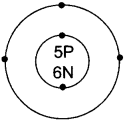What information do you get from the given figure about the atomic number, mass number and valency of the given atom ‘X’:

1. What is the atomic number, the mass number and valency of the atom?
2. Name the element ‘X’.
3. What value of Shivek’s friend are reflected in this behaviour?